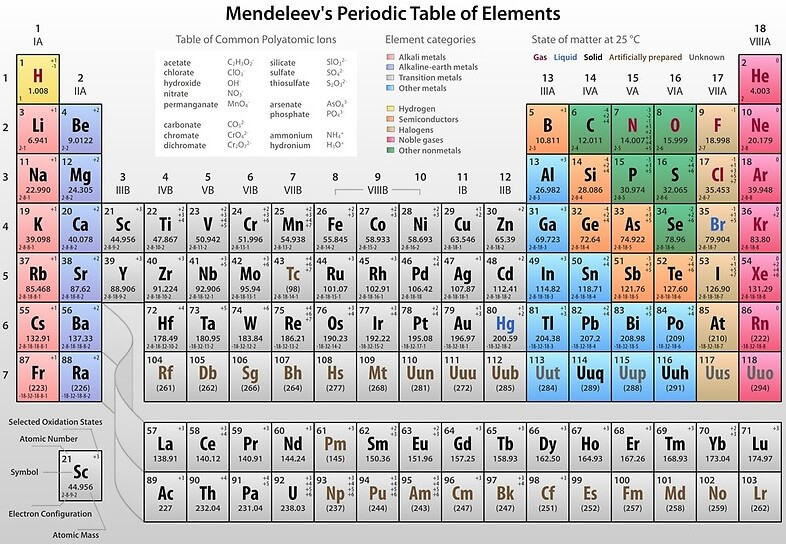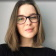# How many valence electrons does lithium have?

My chemistry textbook states that it is 1.
Why is this?
I thought it would be 3, because its electron configuration is: 1s22s1 and 2 + 1 = 3
The second sublevel is not complete.
Can someone explain to me?

﻿
1. Lithium has atomic number of 3. So a neutral lithium atom has 3 protons and 3 electrons. Two electrons in shell 1 and one electron in shell 2. The 1s electrons are ‘core’ electrons.

So Lithium has only 1 valence electron in the 2s orbital.

1. The number of valence electrons in an atom is reflected by its position in the Mendeleev’s periodic table of elements.Across each row, or period, of the periodic table, the number of valence electrons in groups 1-2 and 13-18 increases by one from one element to the next. Within each column, or group, of the table, all the elements have the same number of valence electrons.

Lithium has one valence electron.

1. Lithium (Li) is the third element with a total of 3 electrons. In writing the electron configuration for lithium the first two electrons will go in the 1s orbital. Since 1s can only hold two electrons the remaining electron goes in the 2s orbital. Therefore the Li electron configuration will be 1s22s1.

Li has 1 valence electron.

Psst... Stuck with your assignment? 😱Erica
OnlinePsst... Stuck with your assignment? 😱
Do you need an essay to be done?
Yes
Other assignmentWhat type of assignment 📝 do you need?
• Article review
• Case study
• Coursework
• Report
• Research Paper
• Other AssignmentHow many pages (words) do you need? Let's see if we can help you!